# Avatar Graph

A library for Avatar Graphs.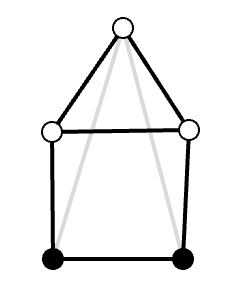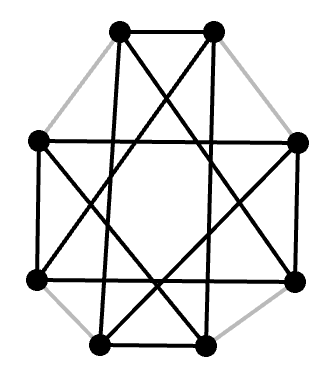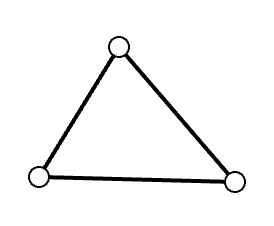An Avatar Graph is a special kind of graph that is useful for mathematical programming.

An Avatar Graph is an sub-type of graphs which is used as a theorem proving technique/tool in Path Semantics.

### Example: Avatar Graphs and Hypercubes

Here is an example of theorem proving with Avatar Graphs.

Conjecture: Any Avatar Graphs containing only core candidates is isomorphic to some hypercube.

If this conjecture is correct, then the number of nodes required to construct a "filled" Avatar Graph is:

`2^n` where `n = 0, 1, 2, ...`.

This conjecture has a counter-proof: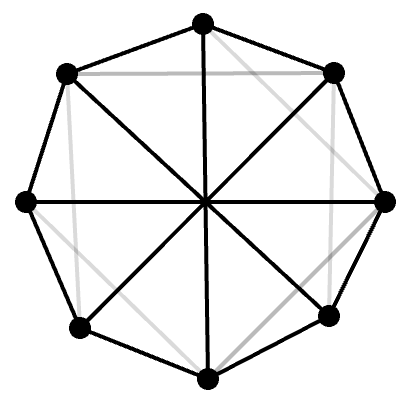This is called a "Wagner graph". See wikipedia article for more information.

It is easier to see that this graph is not isomorphic to a cube, drawn this way: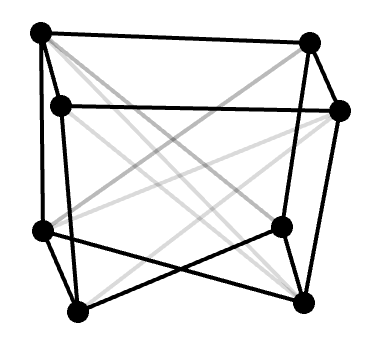However, even the conjecture is wrong, what about the `2^n` law? A Wagner graph has 8 nodes, which is `2^3`! So far, nobody has found a counter-proof to the `2^n` law. This is an open problem.

### Introduction to Avatar Graphs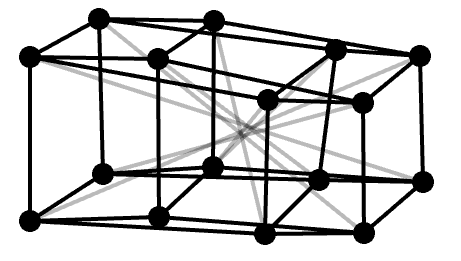A hypercube of 4 dimensions. Any hypercube contains only nodes called "core candidates".

A more practical example for programmers: The New Type Idiom in Rust.

• Why does this technique work?
• How can one prove that such techniques work in general in mathematics?
• How can such techniques be generalized?

It turns out that there is a mathematical pattern behind such generalizations. What these patterns have in common, is that they deal with some kind of encapsulation of information of objects such that they "play a different role", yet they "integrate information" with the original object.

At abstract level, this pattern takes the form of an Avatar Graph.

Avatar Graphs are first and foremost a tool for insight and inspiration. They are usually not needed to be represented explicitly. This means Avatar Graphs can tell something about mathematical theories that is not clear, seen from within the theory.

### Diagrams of Avatar Graphs

The original mathematical model is called the "core self" or just "core" in an Avatar Graph. Avatar Graphs are used to study symmetries of core selfs from avatar extensions.

• black node: Candidate for core self
• white node: N-avatar where where the smallest possible `N` is greater than zero
• black edge: Directed edge depending on choice of core self candidate
• grey/dashed edge: A unique edge from a core self candidate to highest avatar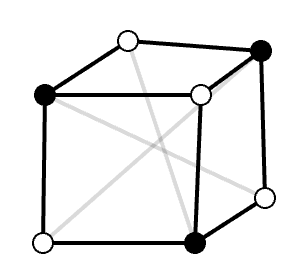### Unique Highest Avatar

Each candidate core has an associated unique highest avatar, due to removal of all irrelevant structure from the graph.

This is because adding new n-avatars at same level is trivial, so it is only interesting to study graphs whith a unique highest avatar.

The choice of highest avatar might depend on order of nodes. This limitation is mostly irrelevant for most interesting graphs. However, it greatly simplifies the calculation of avatar distances.

### Avatar Distances

The `n` in n-avatar is also a kind of distance in the graph, called "avatar distance".

An avatar distance counts the number of paths to the core self.

It is constructed by first finding the shortest distance for all nodes, and then "relaxed" by summing avatar distances of children. The choice of children might depend on the order of nodes.

The exact value of an avatar distance does not matter, except as a mean to find the highest avatar relative to a core candidate.

Think of it of as a somewhat complex puzzle that needs to be solved, and the avatar distances is just a technique for solving the puzzle.

Avatar distances have different semantics than the shortest distance. Two nodes with equal shortest distance can have different avatar distances.

### Avatar Connectivity

Avatars at the same level are not allowed to communicate.

A 1-avatar can only pass information to the core self.

A 2-avatar can only pass information to two 1-avatars.

A 3-avatar can pass information to 3x1-avatars or 2+1-avatars.

An n-avatar can pass information to any partition of lower avatars.

### Non-Contractability

When an avatar has only one child relative to shortest distance, it is contractible, which is not permitted relative to a core candidate.

The core self is not counted as a child for contractability. This means 1-avatars have zero children, therefore not one child. One can also think about it as 1-avatars being an exception from the rule.

### Semi-Contractibility

Since the non-contractibility rule uses shortest distance instead of avatar distances, there are some cases where avatars are semi-contractible.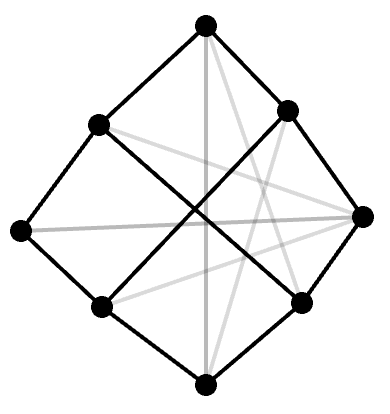Example of a graph with semi-contractibility.

Semi-contractibility happens when avatars are contractible by avatar distance but not by shortest distance.

Semi-contractibility means that two avatars at same level need to agree on how to integrate information. They are using each other as children, depending on the order of nodes in the graph.

This property is allowed since otherwise the Avatar Graphs would not be symmetric in cases where the topology contains features similar to a Möbius strip.

### Universal Reachability

All nodes in the graph must be reachable when walking from the highest avatar to the core candidate, without moving further away than the distance given by the shortest path.

With other words, there exists some path from the highest avatar to any node, while either moving closer, or preserving the distance, to the core candidate.

Another way of thinking about this property, is that for a well-chosen path from the highest avatar to the core, one can visit any node.

This property is beneficial in systems where you want to have choices, but you also want to avoid regression.

## Structs

 Graph Represents an Avatar Graph. Node Represents a node in the graph.